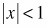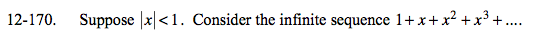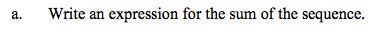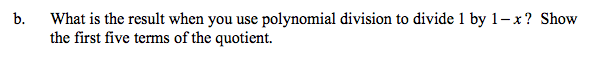### Home > A2C > Chapter 12 > Lesson 12.4.1 > Problem12-170

12-170.
1. Suppose. Consider the infinite sequence 1 + x + x2 + x3 + …. Homework Help ✎

1. Write an expression for the sum of the sequence.

2. What is the result when you use polynomial division to divide 1 by 1 − x? Show the first five terms of the quotient.First find the common ratio, then use the formula for an infinite geometric series.

$\frac{1}{1-x}$Look back at (a) and explore what that answer means.